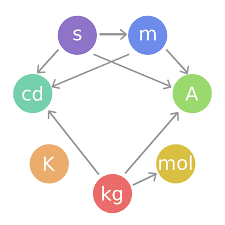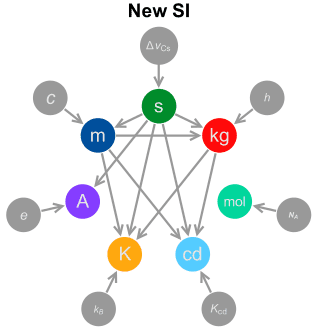# SI Units System

## SI Units System

The International System of Units (SI, abbreviated from the French Système international (d’unités)) is the modern form of the metric system, and is the most widely used system of measurement. It comprises a coherent system of units of measurement built on seven base units (ampere, kelvin, second, metre, kilogram, candela, mole) and a set of twenty decimal prefixes to the unit names and unit symbols that may be used when specifying multiples and fractions of the units. The system also specifies names for 22 derived units for other common physical quantities like lumen, watt, etc.

Base units

The SI base units are the building blocks of the system and all the other units are derived from them. When Maxwell first introduced the concept of a coherent system, he identified three quantities that could be used as base units: mass, length and time. Giorgi later identified the need for an electrical base unit, for which the unit of electric current was chosen for SI. Another three base units (for temperature, amount of substance and luminous intensity) were added later.SI base units Unit name Unit symbol Dimension symbol Quantity name Definition metre m L length Prior (1793): 1/10000000 of the meridian through Paris between the North Pole and the Equator.FG Interim (1960): 1650763.73 wavelengths in a vacuum of the radiation corresponding to the transition between the 2p10 and 5d5 quantum levels of the krypton-86 atom. Current (1983): The distance travelled by light in vacuum in 1/299792458 second. kilogram kg M mass Prior (1793): The grave was defined as being the mass (then called weight) of one litre of pure water at its freezing point.FG Current (1889): The mass of a small squat cylinder of ~47 cubic centimetres of platinum-iridium alloy kept in a laboratory in France. Also, in practice, any of numerous official replicas of it. second s T time Prior: 1/86400 of a day of 24 hours of 60 minutes of 60 seconds Interim (1956): 1/31556925.9747 of the tropical year for 1900 January 0 at 12 hours ephemeris time. Current (1967): The duration of 9192631770 periods of the radiation corresponding to the transition between the two hyperfine levels of the ground state of the caesium-133 atom. ampere A I electric current Prior (1881): A tenth of the electromagnetic CGS unit of current. The [CGS] electromagnetic unit of current is that current, flowing in an arc 1 cm long of a circle 1 cm in radius, that creates a field of one oersted at the centre. Current (1946): The constant current which, if maintained in two straight parallel conductors of infinite length, of negligible circular cross-section, and placed 1 m apart in vacuum, would produce between these conductors a force equal to 2×10−7 newtons per metre of length. kelvin K Θ thermodynamic temperature Prior (1743): The centigrade scale is obtained by assigning 0 °C to the freezing point of water and 100 °C to the boiling point of water. Interim (1954): The triple point of water (0.01 °C) defined to be exactly 273.16 K. Current (1967): 1/273.16 of the thermodynamic temperature of the triple point of water mole mol N amount of substance Prior (1900): A stoichiometric quantity which is the equivalent mass in grams of Avogadro’s number of molecules of a substance. Current (1967): The amount of substance of a system which contains as many elementary entities as there are atoms in 0.012 kilogram of carbon-12. candela cd J luminous intensity Prior (1946): The value of the new candle is such that the brightness of the full radiator at the temperature of solidification of platinum is 60 new candles per square centimetre. Current (1979): The luminous intensity, in a given direction, of a source that emits monochromatic radiation of frequency 5.4×1014 hertz and that has a radiant intensity in that direction of 1/683 watt per steradian. Note: both old and new definitions are approximately the luminous intensity of a whale blubber candle burning modestly bright, in the late 19th century called a “candlepower” or a “candle”.

Notes

• Interim definitions are given here only when there has been a significant difference in the definition.
• Despite the prefix “kilo-“, the kilogram is the base unit of mass. The kilogram, not the gram, is the coherent unit and is used in the definitions of derived units. Nonetheless, prefixes are determined as if the gram were the base unit of mass.
• In 1954 the unit of thermodynamic temperature was known as the “degree Kelvin” (symbol °K; “Kelvin” spelt with an upper-case “K”). It was renamed the “kelvin” (symbol “K”; “kelvin” spelt with a lower case “k”) in 1967.
• When the mole is used, the elementary entities must be specified and may be atoms, molecules, ions, electrons, other particles, or specified groups of such particles.

The Prior definitions of the various base units in the above table were made by the following authorities:

• FG = French Government
• IEC = International Electro Technical Commission
• ICAW = International Committee on Atomic Weights

All other definitions result from resolutions by either CGPM or the CIPM and are cataloged in the SI Brochure.

New SI units System:Derived units

The derived units in the SI are formed by powers, products or quotients of the base units and are unlimited in number. Derived units are associated with derived quantities; for example, velocity is a quantity that is derived from the base quantities of time and length, and thus the SI derived unit is metre per second (symbol m/s). The dimensions of derived units can be expressed in terms of the dimensions of the base units.

Combinations of base and derived units may be used to express other derived units. For example, the SI unit of force is the newton (N), the SI unit of pressure is the pascal (Pa)—and the pascal can be defined as one newton per square metre (N/m2).

 Named SI derived units Namenote 1 Symbol Quantity In other SI units In SI base units radiannote 2 rad angle (m⋅m−1) steradiannote 2 sr solid angle (m2⋅m−2) hertz Hz frequency s−1 newton N force, weight kg⋅m⋅s−2 pascal Pa pressure, stress N/m2 kg⋅m−1⋅s−2 joule J energy, work, heat N⋅m = Pa⋅m3 kg⋅m2⋅s−2 watt W power, radiant flux J/s kg⋅m2⋅s−3 coulomb C electric charge or quantity of electricity s⋅A volt V voltage (electrical potential), emf W/A kg⋅m2⋅s−3⋅A−1 farad F capacitance C/V kg−1⋅m−2⋅s4⋅A2 ohm Ω resistance, impedance, reactance V/A kg⋅m2⋅s−3⋅A−2 siemens S electrical conductance Ω−1 kg−1⋅m−2⋅s3⋅A2 weber Wb magnetic flux V⋅s kg⋅m2⋅s−2⋅A−1 tesla T magnetic flux density Wb/m2 kg⋅s−2⋅A−1 henry H inductance Wb/A kg⋅m2⋅s−2⋅A−2 degree Celsius °C temperature relative to 273.15 K K lumen lm luminous flux cd⋅sr cd lux lx illuminance lm/m2 m−2⋅cd becquerel Bq radioactivity (decays per unit time) s−1 gray Gy absorbed dose (of ionizing radiation) J/kg m2⋅s−2 sievert Sv equivalent dose (of ionizing radiation) J/kg m2⋅s−2 katal kat catalytic activity mol⋅s−1

License: Creative Common share a like